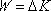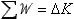This is a big one. It is the work energy theorem (albeit in abbreviated form). The work energy theorem states that the net external work done on a non-deformable object is equal to the change in kinetic energy of that object.

 Notes: 1. Work is a scalar (a number with units) rather than a vector. 2. In using this equation one should actually write it asemphasizing the fact that the net work is the sum of the amount of work done by the individual force acting on the object.

Tip: This is a cause equals effect equation. The cause on the left is equal to the effect on the right. When you use this equation, after first writing it down, you should enter into substitution mode. On the left, substitute the applicable sum of the works for the net external work; on the right, substitute the final kinetic energy minus the initial kinetic energy. Keep the cause on the left and the effect on the right until you have boiled it down to an algebra problem which you can then solve for the sought quantity.